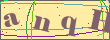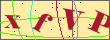# 购车中心

## 询问最低价

*意向车型：

*您的城市：

*您的姓名：

*手机号码：

备　　注：

*验 证 码：## 预约试驾

*意向车型：

*您的城市：

*您的姓名：

*手机号码：

备　　注：

*验 证 码：• 2019.12.16   深圳 苟栋先生 询问最低价成功
• 2019.12.16   昆明 王大锤先生 询问最低价成功
• 2019.12.16   菏泽 胡振先生 询问最低价成功
• 2019.12.16   苏州 胡振先生 预约试驾成功
• 2019.12.16   佛山 朱先生先生 预约试驾成功
• 2019.12.16   邢台 李凡凡女士 询问最低价成功
• 2019.12.16   黄冈 徐昊先生 预约试驾成功
• 2019.12.16   黄冈 梅兴先生 预约试驾成功
• 2019.12.16   三亚 王成先生 预约试驾成功
• 2019.12.16   海南 王成先生 询问最低价成功
﻿
• 快速找车
• 选择品牌
• 选择品牌
• A  奥迪
• A  阿斯顿·马丁
• A  阿尔法·罗密欧
• B  宝沃
• B  布加迪
• B  巴博斯
• B  保时捷
• B  宾利
• B  奔驰
• B  宝马
• B  本田
• B  别克
• B  标致
• B  比亚迪
• B  宝骏
• B  北汽制造
• B  北汽新能源
• B  北汽幻速
• B  北汽威旺
• B  北京汽车
• B  奔腾
• B  北汽绅宝
• C  长安
• C  长安商用
• C  长城
• C  昌河
• D  大众
• D  道奇
• D  DS
• D  东南
• D  东风风神
• D  东风风行
• D  东风小康
• D  东风风度
• D  东风
• F  福特
• F  丰田
• F  菲亚特
• F  法拉利
• F  福田
• F  福迪
• F  福汽启腾
• G  观致
• G  广汽传祺
• G  广汽吉奥
• G  GMC
• H  红旗
• H  汉腾汽车
• H  哈弗
• H  哈飞
• H  海格
• H  海马
• H  华颂
• H  黄海
• H  华泰
• H  恒天
• J  吉利汽车
• J  捷豹
• J  Jeep
• J  江淮
• J  江铃
• J  金杯
• J  九龙
• J  金旅
• K  凯翼
• K  凯迪拉克
• K  克莱斯勒
• K  科尼塞克
• K  卡威
• K  开瑞
• L  路虎
• L  林肯
• L  劳斯莱斯
• L  兰博基尼
• L  雷克萨斯
• L  铃木
• L  雷诺
• L  理念
• L  力帆
• L  莲花汽车
• L  猎豹
• L  路特斯
• L  陆风
• M  马自达
• M  MG
• M  MINI
• M  玛莎拉蒂
• M  摩根
• M  迈凯轮
• N  纳智捷
• O  欧宝
• O  讴歌
• O  欧朗
• Q  奇瑞
• Q  起亚
• Q  启辰
• R  日产
• R  荣威
• R  瑞麒
• S  三菱
• S  斯威汽车
• S  萨博
• S  smart
• S  斯柯达
• S  斯巴鲁
• S  思铭
• S  双龙
• S  上汽大通
• S  双环
• T  特斯拉
• T  腾势
• W  沃尔沃
• W  五菱汽车
• W  五十铃
• W  威兹曼
• W  威麟
• X  现代
• X  雪佛兰
• X  雪铁龙
• X  西雅特
• Y  一汽
• Y  英菲尼迪
• Y  英致
• Y  依维柯
• Y  野马汽车
• Y  永源
• Z  众泰
• Z  中华
• Z  中兴
• Z  知豆
• 选择车系
• 选择车系
• 车型对比
• 选择品牌
• 选择品牌
• A  奥迪
• A  阿斯顿·马丁
• A  阿尔法·罗密欧
• B  宝沃
• B  布加迪
• B  巴博斯
• B  保时捷
• B  宾利
• B  奔驰
• B  宝马
• B  本田
• B  别克
• B  标致
• B  比亚迪
• B  宝骏
• B  北汽制造
• B  北汽新能源
• B  北汽幻速
• B  北汽威旺
• B  北京汽车
• B  奔腾
• B  北汽绅宝
• C  长安
• C  长安商用
• C  长城
• C  昌河
• D  大众
• D  道奇
• D  DS
• D  东南
• D  东风风神
• D  东风风行
• D  东风小康
• D  东风风度
• D  东风
• F  福特
• F  丰田
• F  菲亚特
• F  法拉利
• F  福田
• F  福迪
• F  福汽启腾
• G  观致
• G  广汽传祺
• G  广汽吉奥
• G  GMC
• H  红旗
• H  汉腾汽车
• H  哈弗
• H  哈飞
• H  海格
• H  海马
• H  华颂
• H  黄海
• H  华泰
• H  恒天
• J  吉利汽车
• J  捷豹
• J  Jeep
• J  江淮
• J  江铃
• J  金杯
• J  九龙
• J  金旅
• K  凯翼
• K  凯迪拉克
• K  克莱斯勒
• K  科尼塞克
• K  卡威
• K  开瑞
• L  路虎
• L  林肯
• L  劳斯莱斯
• L  兰博基尼
• L  雷克萨斯
• L  铃木
• L  雷诺
• L  理念
• L  力帆
• L  莲花汽车
• L  猎豹
• L  路特斯
• L  陆风
• M  马自达
• M  MG
• M  MINI
• M  玛莎拉蒂
• M  摩根
• M  迈凯轮
• N  纳智捷
• O  欧宝
• O  讴歌
• O  欧朗
• Q  奇瑞
• Q  起亚
• Q  启辰
• R  日产
• R  荣威
• R  瑞麒
• S  三菱
• S  斯威汽车
• S  萨博
• S  smart
• S  斯柯达
• S  斯巴鲁
• S  思铭
• S  双龙
• S  上汽大通
• S  双环
• T  特斯拉
• T  腾势
• W  沃尔沃
• W  五菱汽车
• W  五十铃
• W  威兹曼
• W  威麟
• X  现代
• X  雪佛兰
• X  雪铁龙
• X  西雅特
• Y  一汽
• Y  英菲尼迪
• Y  英致
• Y  依维柯
• Y  野马汽车
• Y  永源
• Z  众泰
• Z  中华
• Z  中兴
• Z  知豆
• 选择车系
• 选择车系
• 选择车型
• 选择车型
• 意见反馈